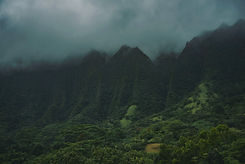top of page# CHEMICAL COMPOSITION

At BattleBlades Inc., we want to be transparent of what goes into the steel we use when forging our products. To further prove that our products truly are museum quality, we tested the chemical composition of each steel we use on our katanas. Tamahagane's composition can be found from its product page.

On the PDF you will find more detailed information about the chemical composition tests.C (carbon)  = 0.58 %
Si (silicon)  = 1.80 %
S (sulfur)  = 0.008 %
P (phosphorus)  =  0.006 %
Mn (manganese)  =  0.79 %
Ni (nickel)  =  0.02 %
Cr (chromium)  =  0.22 %
Mo (molybdenum)  = ＜0.01 %
Cu (copper)  =  0.04 %
W (wolfram/tungsten)  = ＜0.01 %

Clay Tempered  /  Page 2C (carbon)  = 0.68 %
Si (silicon)  = 0.11 %
S (sulfur)  = ＜0.002 %
P (phosphorus)  =  0.014 %
Mn (manganese)  =  0.79 %
Ni (nickel)  =  0.01 %
Cr (chromium)  =  0.66 %
Mo (molybdenum)  = ＜0.01 %
Cu (copper)  =  0.03 %
W (wolfram/tungsten)  = ＜0.01 %

Clay Tempered  /  Page 3C (carbon)  = 0.74 %
Si (silicon)  = 0.17 %
S (sulfur)  = 0.004 %
P (phosphorus)  =  0.022 %
Mn (manganese)  =  0.66 %
Ni (nickel)  =  0.03 %
Cr (chromium)  =  0.19 %
Mo (molybdenum)  =  0.01 %
Cu (copper)  =  0.05 %
W (wolfram/tungsten)  = ＜0.01 %

Clay Tempered  /  Page 4C (carbon)  = 1.05 %
Si (silicon)  = 0.28 %
S (sulfur)  = > 0.002 %
P (phosphorus)  =  0.009 %
Mn (manganese)  =  0.21 %
Ni (nickel)  =  0.13 %
Cr (chromium)  =  0.24 %
Mo (molybdenum)  =  0.06 %
Cu (copper)  =  0.03 %